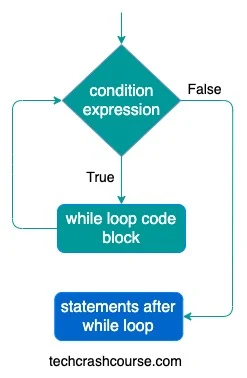# Java While Loop

While loop in java is used to execute a block of code multiple times, until a condition becomes true. Unlike for loop, there is no initialization and increment/decrement step in while loop.

In this comprehensive tutorial, we will delve into the syntax, variations, best practices, and advanced techniques of using the while loop in Java, aiming to equip you with a solid understanding of this essential programming concept.

Here is the syntax of while loop in java
```while(condition_expression) {
// while loop code block
statements;
}
```

condition_expression is a boolean expression which gets evaluated every time before control enters while loop's code block. Here is the description of while loop control flow.

• First of all, while loop evaluates condition_expression inside the parenthesis.
• If condition_expression evaluates to true, then while loop code block gets executed.
• condition_expression is evaluated again.
• This execution process continues until the condition_expression evaluates false.
• Whenever condition_expression evaluates to false, while loop terminates and control goes to the next statement after the while loop.

Here is the flowchart of while loop execution### Java While Loop Example Program

```public class WhileLoop {
public static void main(String[] args) {
int count = 0;
while (count < 5) {
System.out.println(count);
count++;
}
}
}
```
Output
```0
1
2
3
4
```

In above program, while loop executes print statement 5 times, till the time the value of count variable is less than 5. With every iteration the value of count variable is incremented. As soon as value of count becomes 5 while loop terminates.

Here are the execution steps for each iteration of while loop in above program.

Iteration Count Variable Condition
(count < 5)
While Loop Code Block
1 count = 0 true Print count(0).
count is incremented to 1
2 count = 1 true Print count(1).
count is incremented to 2
3 count = 2 true Print count(2).
count is incremented to 3
4 count = 3 true Print count(3).
count is incremented to 4
5 count = 4 true Print count(4).
count is incremented to 5
6 count = 5 false Not Executed

• Nested While Loops : Just like for loops, while loops can also be nested to handle more complex scenarios.
```public class NestedWhileLoopExample {
public static void main(String[] args) {
int outer = 1;

while (outer <= 3) {
int inner = 1;

while (inner <= 3) {
System.out.println("Outer: " + outer + ", Inner: " + inner);
inner++;
}

outer++;
}
}
}
```
In this example, the outer loop executes three times, and for each iteration of the outer loop, the inner loop executes three times, producing a total of nine output lines.

• Infinite While Loop with a Break : You can create an infinite while loop and use a break statement to exit the loop based on a certain condition.
```public class InfiniteWhileLoopExample {
public static void main(String[] args) {
int count = 1;

while (true) {
// Code
count++;

if (count > 5) {
break;
}
}
}
}
```
In this example, the while loop is intentionally infinite, and the break statement is used to exit the loop when the count exceeds 5.

• While Loop for String Manipulation : While loops can be employed for string manipulation tasks, such as reversing a string.
```public class ReverseStringExample {
public static void main(String[] args) {
String original = "Hello, World!";
String reversed = "";

int index = original.length() - 1;

while (index >= 0) {
reversed += original.charAt(index);
index--;
}

System.out.println("Original: " + original);
System.out.println("Reversed: " + reversed);
}
}
```
In this example, the while loop iterates through the characters of the original string in reverse order, creating a reversed string.

## Best Practices for Using While Loops

• Initialize Variables Outside the Loop : Initialize loop control variables outside the while loop to avoid unintentional reinitialization inside the loop.

• Ensure an Exit Condition : Always ensure that there's a clear exit condition to prevent infinite loops. Use a break statement or design the condition such that it becomes false at some point.

• Increment or Decrement Inside the Loop : Increment or decrement loop control variables inside the loop to avoid unintentional infinite loops or skipped iterations

• Use do-while for Post-Condition Checks : If you want to ensure that the loop body is executed at least once, even if the condition is initially false, consider using a do-while loop.

• Keep Loop Body Simple : Keep the body of the while loop simple and avoid complex logic. If the loop body becomes too complex, consider refactoring the code into separate methods.

• Use continue Sparingly : While the continue statement can be useful, excessive use can make the code harder to understand. Consider refactoring the loop or using additional conditional statements to improve clarity.

### Conclusion

The while loop in Java is a versatile and essential construct for repetitive execution of code based on a specified condition. By mastering the basic syntax, understanding variations, adopting best practices, and exploring advanced techniques, you can leverage the full potential of while loops in your Java programs.

As you continue to hone your Java programming skills, practice using while loops in various scenarios. Whether you're iterating over array elements, interacting with user input, or tackling more complex tasks, the while loop is a powerful tool in your programming arsenal. Consistent practice and adherence to best practices will enhance your ability to write efficient, readable, and maintainable code using while loops in Java.